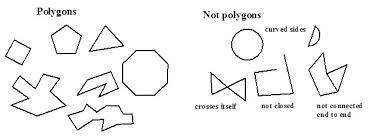# Suppose you are given a polygon. How can you draw a similar figure?

Basic polygons are simple to draw, and you could probably draw a polygon with your eyes closed. Of course if you're given one to copy, it's even easier, although it depends on the type of polygon you are given...A polygon is any shape which encloses a space and is comprised of straight lines, as you see in the examples above. A circle is not a polygon, but triangles, squares, rectangles and so on are polygons. If you need a ruler to draw a shape, it's a polygon!

If a shape intersects itself, it is no longer a simple polygon, but a star polygon, as you see with the 'bow-tie' shape on the right-hand side of the picture. Even so, it can be looked at as two triangles pointing towards each other, and triangles are simple polygons.

The shape you have been given to  may be a many-sided simple polygon, and these have their own names. A five-sided regular polygon is a pentagon (sometimes called a pentagram); a regular six-sided polygon is a hexagon; seven-sided a heptagon, and so on. A regular polygon with 100 sides is a hectogon, and from a distance can look like a circle.

It can be tricky to draw a polygon without being shown how, so grab a pencil, compass and a ruler and watch this YouTube demonstration:

thanked the writer.